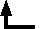## William Thomson, Baron Kelvin of Largs (1824-1907)

### The "Doctrine of Uniformity" in Geology Briefly Refuted.

read before the Royal Society of Edinburgh, December 18, 1865 [from Kelvin, Popular Lectures and Addresses, Vol. II (London: Macmillan, 1894)]

The "Doctrine of Uniformity" in Geology, as held by many of the most eminent of British Geologists, assumes that the earth's surface and upper crust have been nearly as they are at present in temperature, and other physical qualities, during millions of millions of years. But the heat which we know, by observation, to be now conducted out of the earth yearly is so great, that if this action had been going on with any approach to uniformity for 20,000 million years, the amount of heat lost out of the earth would have been about as much as would heat, by 100° Cent., a quantity of ordinary surface rock of 100 times the earth's bulk. (See calculation appended.) This would be more than enough to melt a mass of surface rock equal in bulk to the whole earth. No hypothesis as to chemical action, internal fluidity, effects of pressure at great depth, or possible character of substances in the interior of the earth, possessing the smallest vestige of probability, can justify the supposition that the earth's upper crust has remained nearly as it is, while from the whole, or from any part, of the earth, so great a quantity of heat has been lost.

#### Appendix. Estimate of Present Annual Loss of Heat from the Earth.

Let A be the area of the earth's surface, D the increase of depth in any locality for which the temperature increases by 1° Cent., and k the conductivity per annum of the strata in the same locality. The heat conducted out per annum per square foot of surface in that locality is k/D. Hence, if we give k and D proper average values for the whole upper crust of the earth, the quantity conducted out across the whole earth's surface per annum will be kA/D. The bulk of a sphere being its surface multiplied by 1/3 of its radius, the thermal capacity of a mass of rock equal in bulk to the earth, and of specific heat s per unit of bulk is (1/3)Ars. Hence 3k/(Drs) is the elevation of temperature which a quantity of heat equal to that lost from the earth in a year, would produce in a mass of rock equal in bulk to the whole earth. The laboratory experiments of Peclet; Observations on Underground Temperature in three kinds of rock in and near Edinburgh, by Forbes; in two Swedish strata, by Ångström, and at the Royal Observatory, Greenwich, give values of the conductivity in gramme-water units of heat per square centimetre, per 1° per centimetre of variation of temperature, per second, from .002 (marble, Peclet) to .0107 (sandstone of Craigleith quarry, Forbes); and .005 may be taken as a rough average. Hence, as there are 31,557,000 seconds in a year, we have k = .005 x 31,557,000, or approximately 16x104. The thermal capacity of surface rock is somewhere about half that of equal bulk of water; so that we may take s = .5. And the increase of temperature downwards may be taken as roughly averaging 1° Cent. per 30 metres; so, that, D = 3000 centimetres. Lastly, the earth's quadrant being according to the first foundation of the French metrical system, about 109 centimetres, we may take, in a rough estimate such as the present, r = 6 x 108 centimetres. Hence,
 3k 3x16x104 8 ---------- = ----------------------------------- = -------------- Drs 3000x6x108x.5 15x106
This, multiplied by 20,000 x 106, amounts to 10,000, or to 100 times as much heat as would warm 10 times the earth's bulk of surface rock by 1° Cent.Back to the list of selected historical papers.Back to the top of Classic Chemistry.